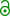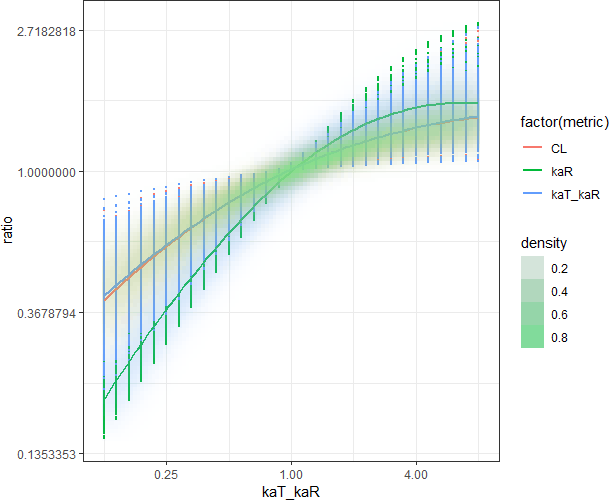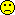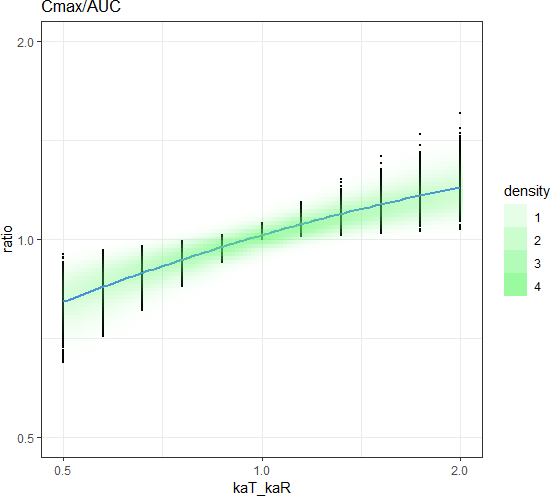Helmut
★★★Vienna, Austria,
2019-04-15 12:08

Posting: # 20163
Views: 3,908

## Metrics for absorption [NCA / SHAM]

Dear all,

interesting read hot off the press:

Vincze I, Endrényi L, Tóthfalusi L. Bioequivalence metrics for absorption rates: linearity, specificity, sensitivity. Acta Pharm Hung. 2019;89(1):17–21. doi:10.33892/aph.2019.89.17-21.free resource.

Dif-tor heh smusma 🖖
Helmut SchützThe quality of responses received is directly proportional to the quality of the question asked. 🚮
Science Quotes
nobody
nothing

2019-04-15 12:15

@ Helmut
Posting: # 20164
Views: 3,696

## Metrics for absorption

Hi

first thought

"Cmax is ...is nonspecific to it (reflects also the extent of absorption as well as the rates of disposition processes),..."

Hmm, how is AUCp not reflecting disposition processes?

"...lacks kinetic sensitivity even following a single administration."

How much sensitivity is needed in the first place? Or are we creating problems by "overly sensitive" methods with no relevance in clinical practice?

Kindest regards, nobody
ElMaestro
★★★

Belgium?,
2019-04-15 12:23

@ nobody
Posting: # 20165
Views: 3,677

## Metrics for absorption

Hi all,

terribly sorry to ask this, but what is disposition really, as in really-really ??

I am not asking for a link or C&P to some more or less half-assed or vague definition somewhere, but hopefully someone in his/her own words can tell me exactly what disposition means in the context of this paper or how the term generally should be understood when we discuss PK?

I could be wrong, but...

Best regards,
ElMaestro

"Pass or fail" (D. Potvin et al., 2008)
nobody
nothing

2019-04-15 12:39

@ ElMaestro
Posting: # 20166
Views: 3,683

## Metrics for absorption

Disposition = Distribution + Clearance

Kindest regards, nobody
Helmut
★★★Vienna, Austria,
2019-04-15 13:02

@ nobody
Posting: # 20167
Views: 3,677

## Metrics for absorption

Hi nobody,

» "Cmax is ...is nonspecific to it (reflects also the extent of absorption as well as the rates of disposition processes),..."
»
» Hmm, how is AUCp not reflecting disposition processes?

It is as well. In the ideal case the sensitivity of a metric would be 1.
See Table I: Both are lousy but pAUC less so.

» "...lacks kinetic sensitivity even following a single administration."
»
» How much sensitivity is needed in the first place?

Duno. If we have different metrics likely it would be better to chose one with high sensitivity. I’m surprised that they didn’t explore Cmax/AUC which was shown by the two Lászlós1,2 and Lacey et al.3,4 to be superior to Cmax. Also interesting the close relationship between tmax and Cmax/AUC.5,6 My simulations are running…

» Or are we creating problems by "overly sensitive" methods with no relevance in clinical practice?

IMHO, the clinical relevance of the rate of absorption is doubtful. Ask a clinician about Cmax and he will tell you that is important for safety, being not aware that Emax is the trigger. Emax is linked to Cmax with a sometimes complicated function but generally dampened. If I’m interested in rapid onset I would assess tmax. But that’s another story.
Remember that current regulatory thinking concentrates on the biopharmaceutical performance of the formulation in the first place. Mentioning clinical relevance outs you as a dinosaur of BE.PS: Please don’t post in all capital letters. THX.

1. Endrényi L, Fritsch S, Yan W. Cmax/AUC is a clearer measure than Cmax for absorption rates in investigations of bioequivalence. Int J Clin Pharmacol Ther Toxicol. 1991;29(10):394-9.
2. Tóthfalusi L, Endrényi L. Without extrapolation, Cmax/AUC is an effective metric in investigations of bioequivalence. Pharm Res. 1995;12(6):937-42. doi:10.1023/A:1016237826520
3. Lacey LF, Keene ON, Duquesnoy C. Evaluation of different indirect measures of rate of drug absorption in pharmacokinetic studies. 5th European Congress of Biopharmaceutics and Pharmacokinetics, Brussels, Belgium; 21 April 1993:P273.
4. Lacey LF, Keene ON, Bye E. Evaluation of Different Metrics as Indirect Measures of Rate of Drug Absorption. In: Blume HH, Midha KK, editors. Bio-International 2. Bioavailability, Bioequivalence and Pharmacokinetic Studies. Stuttgart: medpharm; 1995. p. 73–85.
5. Schall R, Luus HG. Comparison of absorption rates in bioequivalence studies of immediate release drug formulations. Int J Clin Pharmacol Ther Toxicol. 1992;30(5):153–9.
6. Schall R, Luus HG, Steinijans VW, Hauschke D. Choice of characteristics and their bioequivalence ranges for the comparison of absorption rates of immediate-release drug formulations. Int J Clin Pharmacol Ther. 1994;32(7):323-8.

Dif-tor heh smusma 🖖
Helmut SchützThe quality of responses received is directly proportional to the quality of the question asked. 🚮
Science Quotes
nobody
nothing

2019-04-15 13:48
(edited by nobody on 2019-04-15 17:01)

@ Helmut
Posting: # 20168
Views: 3,663

## Metrics for absorption

» Hi nobody,

Ho Vienna

» If we have different metrics likely it would be better to chose one with high sensitivity.

Not more than needed. Over-discriminatory testing is a pain. I like the idea of "problem -> solution", not the other way around.

» IMHO, the clinical relevance of the rate of absorption is doubtful. Ask a clinician about Cmax and he will tell you that is important for safety,

Safety is part of clinics as well. If your patients die from toxicity or headache or bleeding or whatever you will learn the hard way.

» Remember that current regulatory thinking concentrates on the biopharmaceutical performance of the formulation in the first place. Mentioning clinical relevance outs you as a dinosaur of BE.Yeah, but in the end you are not interested in differences in "biopharm. performance" not reflecting any meaningful (= clinically relevant) differences between two formulations, or?

Kindest regards, nobody
Helmut
★★★Vienna, Austria,
2019-04-15 19:31

@ nobody
Posting: # 20169
Views: 3,624

## Metrics for absorption

Hi nobody,

» » If we have different metrics likely it would be better to chose one with high sensitivity.
»
» Not more than needed. Over-discriminatory testing is a pain. I like the idea of "problem -> solution", not the other way around.

OK. Define what is “needed”. Reminds me on mathematicians’ “sufficiently accurate”. There is an exact solution of the three-body problem but it converges so slow that one would need an infinite number of terms.» » IMHO, the clinical relevance of the rate of absorption is doubtful. Ask a clinician about Cmax and he will tell you that is important for safety,
»
» Safety is part of clinics as well. If your patients die from toxicity or headache or bleeding or whatever you will learn the hard way.

Sure. I only meant that the relationship Cmax = safety is an oversimplification.

» » Remember that current regulatory thinking concentrates on the biopharmaceutical performance of the formulation in the first place. Mentioning clinical relevance outs you as a dinosaur of BE.»
» Yeah, but in the end you are not interested in differences in "biopharm. performance" not reflecting any meaningful (= clinically relevant) differences between two formulations, or?

Absolutely. However, that similarity of PK (in healthy subjects) can be extrapolated to the population of patients is based on an assumption. The days were BE was tested in settings closer to the clinical situation are long gone – which regret.
• If for chronic use, only in steady state.
• If t½ of active metabolite > t½ of parent, assess only the metabolite.
I don’t like that but in this century biopharmaceutical performance is all what counts for regulators.

The more I think about this paper, the less I understand it. Not the first time with one the two Lászlós. ke in their simulations is a constant. Since we compare T/R I would assess the impact of a set of absorption constants (different Test) to the ka of R. Code at the end. I got

  kaR kaT_kaR     kaT  Cmax Cmax.r   pAUC pAUC.r Cmax_AUC Cmax_AUC.r  1.39   12.00 16.6800 368.4 1.4620 414.40 1.6680   0.3248     1.4540  1.39    8.00 11.1200 357.7 1.4190 406.50 1.6360   0.3154     1.4120  1.39    4.00  5.5600 332.6 1.3190 381.70 1.5360   0.2934     1.3140  1.39    2.00  2.7800 297.3 1.1790 330.60 1.3310   0.2626     1.1760  1.39    1.00  1.3900 252.1 1.0000 248.50 1.0000   0.2233     1.0000  1.39    0.50  0.6950 200.1 0.7939 160.40 0.6457   0.1791     0.8021  1.39    0.25  0.3475 147.3 0.5842  92.62 0.3728   0.1389     0.6219

where kaR = ka(R), kaT_kaR = ka(T)/ka(R), kaT = ka(T), Cmax.r = Cmax(T)/Cmax(R), pAUC.r = pAUC(T)/pAUC(R), and Cmax_AUC.r = [Cmax(T)/AUC(T)]/Cmax(T)/AUC(T)]. Since F = 1 in all cases and ke is the same so is the AUC. I would say an ideal metric should reflect changes in ka in the T/R-ratio, or? Given my results all are lousy but Cmax the least. Either I screwed up the code or completely misunderstood the two Lászlós.

library("colorspace") C.sd <- function(F=1, D, Vd, ka, ke, t) {   if (!identical(ka, ke)) { # common case ka != ke     C <- F*D/Vd*(ka/(ka - ke))*(exp(-ke*t) - exp(-ka*t))   } else {                  # equal input & output     C <- F*D/Vd*ke*t*exp(-ke*t)   }   return(C) } D        <- 400 ka       <- 1.39  # 1/h Vd       <- 1     # L CL       <- 0.347 # L/h ke       <- CL/Vd t        <- seq(0, 12, length.out=2000) # small step size to minimize the                                         # intrinsic bias of the trapezoidal # Reference C        <- C.sd(D=D, Vd=Vd, ka=ka, ke=ke, t=t) tmax     <- log((ka/ke)/(ka - ke)) # theoretical /not/ observed Cmax     <- C.sd(D=D, Vd=Vd, ka=ka, ke=ke, t=tmax) AUC.t    <- 0.5*sum(diff(t)*(C[-1]+C[-length(C)])) t.1      <- t[which(t <= tmax)] t.cut    <- max(t.1) C.1      <- C[which(t <= t.cut)] pAUC     <- 0.5*sum(diff(t.1)*(C.1[-1]+C.1[-length(C.1)])) Cmax.AUC <- Cmax/AUC.t dev.new(record=TRUE) plot(t, C, type="n", las=1, lwd=5, col="red", ylim=c(0, 375),      xlab="Time (h)", ylab="Concentration (mg/mL)") grid() abline(v=tmax, lty=3, col="grey50") lines(t, C, lwd=6, col="red") # Tests ratio <- c(seq(12, 4, -4), 2, 1, 0.5, 0.25) # ratios of ka(T)/ka/R) ka.t  <- ka*ratio                           # Tests' ka res   <- data.frame(kaR=ka, kaT_kaR=ratio, kaT=signif(ka.t, 5),                     Cmax=NA, Cmax.r=NA, pAUC=NA, pAUC.r=NA,                     Cmax_AUC=NA, Cmax_AUC.r=NA) clr   <- sequential_hcl(length(ratio), palette="Red-Blue") for (j in seq_along(ratio)) {   # full internal precision, 4 significant digits for output   C.tmp    <- C.sd(D=D, Vd=Vd, ka=ka.t[j], ke=ke, t=t)   if (!identical(ka.t[j], ke)) { # ka != ke     tmax.tmp <- log(ka.t[j]/ke)/(ka.t[j] - ke)   } else {                       # ka = ke     tmax.tmp <- 1/ke   }   Cmax.tmp <- C.sd(D=D, Vd=Vd, ka=ka.t[j], ke=ke, t=tmax.tmp)   res[j, "Cmax"]   <- signif(Cmax.tmp, 4)   res[j, "Cmax.r"] <- signif(Cmax.tmp/Cmax, 4)   AUC.t.tmp <- 0.5*sum(diff(t)*(C.tmp[-1]+C.tmp[-length(C.tmp)]))   t.1.tmp   <- t[which(t <= t.cut)]   C.1.tmp   <- C.tmp[which(t <= t.cut)] # cut at tmax of R!   pAUC.tmp  <- 0.5*sum(diff(t.1.tmp)*(C.1.tmp[-1]+C.1.tmp[-length(C.1.tmp)]))   res[j, "pAUC"]       <- signif(pAUC.tmp, 4)   res[j, "pAUC.r"]     <- signif(pAUC.tmp/pAUC, 4)   res[j, "Cmax_AUC"]   <- signif(Cmax.tmp/AUC.t.tmp, 4)   res[j, "Cmax_AUC.r"] <- signif((Cmax.tmp/AUC.t.tmp)/Cmax.AUC, 4)   lines(t, C.tmp, lwd=2, col=clr[j]) } legend("topright", legend=res$kaT_kaR, bg="white", inset=0.02, title="ka(T) / ka(R)", lwd=2, col=clr) plot(ratio, res$Cmax.r, type="n", log="xy", las=1, col="red",      ylim=range(c(res$Cmax.r, res$pAUC.r, res$Cmax_AUC.r)), xlab="ka (T) / ka (R)", ylab="Metric ratio") grid() abline(v=1, lty=3, col="grey50") abline(h=1, lty=3, col="grey50") lines(ratio, res$Cmax.r, lwd=2, col="red") lines(ratio, res$pAUC.r, lwd=2, col="blue") lines(ratio, res$Cmax_AUC.r, lwd=2, col="magenta") legend("bottomright", legend=c("Cmax", "pAUC", "Cmax/AUC"),        bg="white", inset=0.02, title="T/R-ratio", lwd=2,        col=c("red", "blue", "magenta")) cat("x.r is the T/R-ratio of metric x\n");print(res, row.names=FALSE)

Dif-tor heh smusma 🖖
Helmut SchützThe quality of responses received is directly proportional to the quality of the question asked. 🚮
Science Quotes
nobody
nothing

2019-04-16 09:34
(edited by nobody on 2019-04-16 10:39)

@ Helmut
Posting: # 20172
Views: 3,616

## Metrics for absorption

...maybe I got you completely wrong, but for me ka ratio varies 48-fold, while cmax ratio only 2.50-fold, pAUC 4.47-fold and cmax/AUC 2.34-fold over the range tested.

So pAUC is indeed the best of all-not-so-wonderful parameters?

Kindest regards, nobody
nobody
nothing

2019-04-16 15:15

@ nobody
Posting: # 20174
Views: 3,582

## Metrics for absorption

PS: How about calculating the slope from at least 3 points before cmax (excluding cmax) and calling it, let's say, "invasion constant", which should be (if CL is constant) a measure for ka, or?

Kindest regards, nobody
Helmut
★★★Vienna, Austria,
2019-04-16 17:01

@ nobody
Posting: # 20176
Views: 3,570

## Metrics for absorption

Hi nobody,

method of residuals, feathering, Wagner-Nelson? Smells of modeling which is not acceptable in BE. BTW, lag-times are the killer. Good luck!1,2

1. Nerella NG, Block NH, Noonan PK. The Impact of Lag Time on the Estimation of Pharmacokinetic Parameters. I. One Compartment Model. Pharm Res. 1993;10(7):1031–6.
2. Csizmadia F, Endrényi L. Model-Independent Estimation of Lag Times with First-Order Absorption and Disposition. J Pharm Sci. 1998;87(5):608–12. doi:10.1021/js9703333

Dif-tor heh smusma 🖖
Helmut SchützThe quality of responses received is directly proportional to the quality of the question asked. 🚮
Science Quotes
nobody
nothing

2019-04-17 11:15

@ Helmut
Posting: # 20182
Views: 3,448

## Metrics for absorption

Hi Helmut,

simple linear regression, only consider points from, let's say, 15% of Cmax to time point of Cmax-1 (i.e. excluding Cmax from regression). Would need data from 100 different compounds real-life data for the start...

Kindest regards, nobody
mittyri
★★

Russia,
2019-04-17 01:21

@ Helmut
Posting: # 20179
Views: 3,582

## Simulation framework

Hi Helmut,

» If t½ of active metabolite > t½ of parent, assess only the metabolite.

Could you please explain a little bit? When did I miss that good old times?

library(ggplot2) # input paraemeters Nsub <- 1000 # number of subjects to simulate D        <- 400 ka       <- 1.39  # 1/h ka.omega <- 0.1 Vd       <- 1     # L Vd.omega <- 0.2 CL       <- 0.347 # L/h CL.omega <- 0.15 t<- c(seq(0, 1, 0.25), seq(2,6,1), 8,10,12,16,24) # some realistic sequence ratio <- 2^(seq(-3,3,0.2)) # ratios of ka(T)/ka/R) # helper functions C.sd <- function(F=1, D, Vd, ka, ke, t) {   if (!identical(ka, ke)) { # common case ka != ke     C <- F*D/Vd*(ka/(ka - ke))*(exp(-ke*t) - exp(-ka*t))   } else {                  # equal input & output     C <- F*D/Vd*ke*t*exp(-ke*t)   }   return(C) } AUCcalc <- function(t,C){   linlogflag <- C[-length(C)] <= C[-1]   AUCsegments <- ifelse(linlogflag,                    diff(t)*(C[-1]+C[-length(C)])/2,                    (C[-length(C)] - C[-1])*diff(t)/(log(C[-length(C)]) - log(C[-1])))   return(sum(AUCsegments)) } AbsorptionDF <- function(D, ka, Vd, CL,t,ratio){   # Reference   ke       <- CL/Vd   C        <- C.sd(D=D, Vd=Vd, ka=ka, ke=ke, t=t)   tmax     <- t[C == max(C)]   Cmax     <- C.sd(D=D, Vd=Vd, ka=ka, ke=ke, t=tmax)   AUC.t    <- AUCcalc(t, C)   t.1      <- t[which(t <= tmax)]   t.cut    <- max(t.1)   C.1      <- C[which(t <= t.cut)]   pAUC     <- AUCcalc(t.1, C.1)   Cmax.AUC <- Cmax/AUC.t     # Tests   ka.t  <- ka*ratio                           # Tests' ka   res   <- data.frame(kaR=ka, kaT_kaR=ratio, kaT=signif(ka.t, 5),                       Cmax=NA, Cmax.r=NA, pAUC=NA, pAUC.r=NA,                       Cmax_AUC=NA, Cmax_AUC.r=NA)     for (j in seq_along(ratio)) {     # full internal precision, 4 significant digits for output     C.tmp    <- C.sd(D=D, Vd=Vd, ka=ka.t[j], ke=ke, t=t)     if (!identical(ka.t[j], ke)) { # ka != ke       tmax.tmp <- log(ka.t[j]/ke)/(ka.t[j] - ke)     } else {                       # ka = ke       tmax.tmp <- 1/ke     }     Cmax.tmp <- C.sd(D=D, Vd=Vd, ka=ka.t[j], ke=ke, t=tmax.tmp)     res[j, "Cmax"]   <- signif(Cmax.tmp, 4)     res[j, "Cmax.r"] <- signif(Cmax.tmp/Cmax, 4)     AUC.t.tmp <- AUCcalc(t,C.tmp)     t.1.tmp   <- t[which(t <= t.cut)]     C.1.tmp   <- C.tmp[which(t <= t.cut)] # cut at tmax of R!     pAUC.tmp  <- AUCcalc(t.1.tmp, C.1.tmp)     res[j, "pAUC"]       <- signif(pAUC.tmp, 4)     res[j, "pAUC.r"]     <- signif(pAUC.tmp/pAUC, 4)     res[j, "Cmax_AUC"]   <- signif(Cmax.tmp/AUC.t.tmp, 4)     res[j, "Cmax_AUC.r"] <- signif((Cmax.tmp/AUC.t.tmp)/Cmax.AUC, 4)   }   return(res) } SubjectsDF <- data.frame() for(isub in 1:Nsub){   # sampling parameters   ka.sub       <- ka * exp(rnorm(1, sd = sqrt(ka.omega)))   Vd.sub       <- Vd * exp(rnorm(1,sd = sqrt(Vd.omega)))   CL.sub       <- CL * exp(rnorm(1,sd = sqrt(CL.omega)))   DF.sub <- cbind(Subject = isub, V = Vd.sub, CL = CL.sub, AbsorptionDF(D, ka.sub, Vd.sub, CL.sub, t, ratio))   SubjectsDF <- rbind(SubjectsDF, DF.sub) } SubjectsDFstack <-   reshape(SubjectsDF[, -c(2,3,4,6,7,9,11)],         direction = 'long', varying = 3:5, v.names = "ratio", timevar = "metric", times = names(SubjectsDF)[3:5]) # hate this one! ggplot(SubjectsDFstack, aes(x=kaT_kaR, y=ratio, color=factor(metric)) ) +   theme_bw() +   geom_point(size=.3) +   geom_smooth(method = 'loess', se = FALSE) +   stat_density_2d(data = subset(SubjectsDFstack, metric == unique(SubjectsDFstack$metric)), geom = "raster", aes(alpha = ..density..), fill = "#F8766D" , contour = FALSE) + stat_density_2d(data = subset(SubjectsDFstack, metric == unique(SubjectsDFstack$metric)), geom = "raster", aes(alpha = ..density..), fill = "#6daaf8" , contour = FALSE) +   stat_density_2d(data = subset(SubjectsDFstack, metric == unique(SubjectsDFstack$metric)), geom = "raster", aes(alpha = ..density..), fill = "#6df876" , contour = FALSE) + scale_alpha(range = c(0, 0.7)) + scale_x_continuous(trans='log2') + scale_y_continuous(trans='log')Kind regards, Mittyri Helmut ★★★Vienna, Austria, 2019-04-17 13:31 @ mittyri Posting: # 20183 Views: 3,475 ## Simulation framework Hi mittyri, » » If t½ of active metabolite > t½ of parent, assess only the metabolite. » » Could you please explain a little bit? When did I miss that good old times?This was the standard approach till the mid 1990s.1,2,3 The idea behind was clinical relevance. An example often discussed at that time was amitriptyline (t½ ~16 h) and its main metabolite nortriptyline (t½ >30 h). Both are about equally potent. In steady state (you never ever administer a single dose of a tricyclic antidepressant) the metabolite causes >⅔ of the effect. Which one is more relevant? Tucker even argued that in a linear system any compound with the lowest variability (parent, active or inactive metabolite) could be chosen. Hence, for a while he was called Geoff “pick-out-the-best” Tucker in the community. Already at the Bio-International in 1994 the pendulum started to swing towards the approach we are now bound to.4 Since its frequency is low and its amplitude high we will have to wait a good while till it turns.BTW, proceedings of the Bio-International conferences make still a great read and help in understanding how – and why – we ended up here.© Tomas Salmonson Maybe you can get the first ones used. If you find the third covering the Bio-International 1996 in Yokohama somewhere let me know. I lost mine… » ready for simulation: I corrected a typo in your original post from » SubjectsDFstack <- reshape(SubjectsDF[, -c(2,3,4,6,7,9,11)], direction = 'long', varying = 3:5, v.names = "ratio", timevar = "metric", times = names(SubjectsDF1)[3:5]) to SubjectsDFstack <- reshape(SubjectsDF[, -c(2,3,4,6,7,9,11)], direction = 'long', varying = 3:5, v.names = "ratio", timevar = "metric", times = names(SubjectsDF)[3:5]) So what do you conclude? 1. Importance of Metabolites in Assessment of Bioequivalence. In: Midha KK, Blume HH, editors. Bio-International. Bioavailability, Bioequivalence and Pharmacokinetics. Stuttgart; medpharm: 1993. p. 147–208. 2. Blume HH, Midha KK. Bio-International ’92, Conference on Bioavailability, Bioequivalence and Pharmacokinetic Studies. Pharm Res.1993;10(12):1806–11. doi:10.1023/A:1018998803920. 3. Tucker GT. Bioequivalence – A Measure of Therapeutic Equivalence? In: Blume HH, Midha KK, editors. Bio-International 2. Bioavailability, Bioequivalence and Pharmacokinetic Studies. Stuttgart; medpharm: 1995. p. 35–43. 4. Welling PG. Bioequivalence – A Measure of Quality Control? In: Blume HH, Midha KK, editors. Bio-International 2. Bioavailability, Bioequivalence and Pharmacokinetic Studies. Stuttgart; medpharm: 1995. p. 45–49. Dif-tor heh smusma 🖖 Helmut SchützThe quality of responses received is directly proportional to the quality of the question asked. 🚮 Science Quotes mittyri ★★ Russia, 2019-04-17 15:42 @ Helmut Posting: # 20184 Views: 3,394 ## Conclusion Hi Helmut, thank you so much for comrehensive answer! » I corrected a typo in your original post forgot to execute it on clean environment, sorry! » So what do you conclude? pAUC is more sensitive, but the sensitivity is still low (nothing new...) Kind regards, Mittyri Helmut ★★★Vienna, Austria, 2019-04-17 17:05 @ mittyri Posting: # 20185 Views: 3,411 ## Conclusion & beyond Hi mittyri, » » I corrected a typo in your original post » » forgot to execute it on clean environment, sorry! Welcome to the club! BTW, THX for implementing the lin-up/log-down trapezoidal.» » So what do you conclude? » » pAUC is more sensitive, but the sensitivity is still low (nothing new...) Yep. Actually this story reaches too far (for an IR formulation crossing flip-flop PK; no regulator would buy that regardless what is written in a guideline) and not far enough: Setting the cut-off for pAUC at the individual tmax-values of the reference is Canadian tradition (termed AUCReftmax) but even in Canada history. If pAUCs are used, the cut-off has to be pre-specified. Currently pAUCs are not required for IR. OK, if the first pAUC performs better than Cmax, great. I think that limiting ka(T)/ka(R) to 0.5–2 is sufficient. For the cut-off 2×tmax=2log(ka/ke/(ka–ke)), what else? Edit: No wonder you hated this line of your code. Shouldn’t it be: SubjectsDFstack <- reshape(SubjectsDF[, -c(2,3,4,6,7,9,11)], direction = 'long', varying = c(3:5), v.names = "ratio", timevar = "metric", times = names(SubjectsDF)[c(7,9,11)]) My sim’s: In the input section t.cut <- 2*log(ka/(CL/Vd)/(ka-(CL/Vd))) Then (relevant lines only): AbsorptionDF <- function(D, ka, Vd, CL,t,ratio,t.cut){ # Reference ke <- CL/Vd C <- C.sd(D=D, Vd=Vd, ka=ka, ke=ke, t=t) tmax <- t[C == max(C)] Cmax <- C.sd(D=D, Vd=Vd, ka=ka, ke=ke, t=tmax) AUC.t <- AUCcalc(t, C) t.1 <- t[which(t <= t.cut)] C.1 <- C[which(t <= t.cut)] pAUC <- AUCcalc(t.1, C.1) Cmax.AUC <- Cmax/AUC.t DF.sub <- cbind(Subject = isub, V = Vd.sub, CL = CL.sub, AbsorptionDF(D, ka.sub, Vd.sub, CL.sub, t, ratio, t.cut)) sp1 <- ggplot(SubjectsDFstack[SubjectsDFstack$metric == "Cmax", ],               aes(x=kaT_kaR, y=ratio, color=factor(metric))) sp1 + theme_bw() +   geom_point(size=.3) +   geom_smooth(method = 'loess', se = FALSE) +   stat_density_2d(data = SubjectsDFstack[SubjectsDFstack$metric == "Cmax", ], geom = "raster", aes(alpha = ..density..), fill = "#F8766D", contour = FALSE) + scale_alpha(range = c(0, 0.7)) + scale_x_continuous(trans='log2') + scale_y_continuous(limits=c(0.5,2), trans='log2') sp2 <- ggplot(SubjectsDFstack[SubjectsDFstack$metric == "pAUC", ],               aes(x=kaT_kaR, y=ratio, color=factor(metric))) sp2 + theme_bw() +   geom_point(size=.3) +   geom_smooth(method = 'loess', se = FALSE) +   stat_density_2d(data = SubjectsDFstack[SubjectsDFstack$metric == "pAUC", ], geom = "raster", aes(alpha = ..density..), fill = "#6DAAF8", contour = FALSE) + scale_alpha(range = c(0, 0.7)) + scale_x_continuous(trans='log2') + scale_y_continuous(limits=c(0.5,2), trans='log2') sp3 <- ggplot(SubjectsDFstack[SubjectsDFstack$metric == "Cmax_AUC", ],               aes(x=kaT_kaR, y=ratio, color=factor(metric))) sp3 + theme_bw() +   geom_point(size=.3) +   geom_smooth(method = 'loess', se = FALSE) +   stat_density_2d(data = SubjectsDFstack[SubjectsDFstack\$metric == "Cmax_AUC", ],     geom = "raster", aes(alpha = ..density..), fill = "#6DF876",     contour = FALSE) +   scale_alpha(range = c(0, 0.7)) +   scale_x_continuous(trans='log2') +   scale_y_continuous(limits=c(0.5,2), trans='log2')Based on loess:
   metric kaT_kaR predicted sensitivity  Cmax_AUC     0.5 0.8082842  0.54181715      Cmax     0.5 0.8075275  0.54298163      pAUC     0.5 0.7143879  0.76337666      pAUC     2.0 1.2181904  0.08875421  Cmax_AUC     2.0 1.2012353  0.10671423      Cmax     2.0 1.2028693  0.10745301

Again, pAUC is the one-eyed leading the blind ones but only if kaT < kaR.

Dif-tor heh smusma 🖖
Helmut SchützThe quality of responses received is directly proportional to the quality of the question asked. 🚮
Science QuotesIng. Helmut Schütz